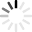# Right Triangle Quizzes & Trivia

Don’t go left with this one! Carpenters, surveyors, and architects use this on a daily basis to build things essential for everyone such as buildings, chairs, and tables. We promise that you will have a blast with our quizzes about right triangles! Protract your brain with questions like: “What is a hypotenuse?”, “What is the Pythagorean Theroem why is it important?”, “What is Thales’ theorem and why is it important?”, “What is a special right triangle?”, and “What is the formula for calculating areas of right triangles?”.

This quizzes will give you 90 degrees of fun. Always remember to give your best. Good luck and just like a right triangle our quizzes are the right choice.
Top Trending

A right triangle is a triangle in which one angle is a right angle. The relation between the sides and angles of a right triangle is the basis for trigonometry. For practice on this and more, take this quiz.

Questions: 9  |  Attempts: 713   |  Last updated: Nov 9, 2017
• Sample Question
For the right triangle ABC shown below, what is tanA?Students will test their knowledge on 30-60-90 and 45-45-90 triangles.

Questions: 5  |  Attempts: 574   |  Last updated: May 1, 2017
• Sample Question
Find x.Pythagorean Theorem and its converse; Special Right Triangles; Trigonometry

Questions: 15  |  Attempts: 355   |  Last updated: Jan 24, 2013
• Sample Question
Rita drew right triangle GHK on a sheet of notebook paper.  ___ GH  = 3 cm (LL) ___ GH  = 5 cm (Hypotenuse) To the nearest centimeter, what is the perimeter of the triangle?Questions: 5  |  Attempts: 226   |  Last updated: Feb 3, 2014
• Sample Question
In a right angle triangle ABC, AB = 6 cm, AC = 10 cm and angle ABC = 90 degrees. The length of side BC isThis is your chapter test for section 4 on right triangles.  Please answer the questions on your own without the use of notes.  You may use your Formulas Quiz from Tuesday.  You may only take the exam once.

Questions: 35  |  Attempts: 58   |  Last updated: Jan 22, 2013
• Sample Question
A right triangle has two legs of lengths 4, and 8.  What is the length of the hypotenuse?Related